BASH Programming

# Find Length of String in Bash

The total number of characters of any string data indicates the length of the string. When we work with string data then it is important to count the length of the string for various programming tasks. The built-in function exists to count the total number of characters in many programming languages. But bash has no this type of built-in function. The length of the string can be counted in bash in multiple ways. How you can find out the length of a string data in bash is shown in this tutorial by using different examples.

## Syntax:

Any of the following syntaxes can be followed to count the length of string.

\${#strvar}
expr length \$strvar
expr\${strvar}”:’.*
\$strvar | wc -c
\$strvar |awk '{print length}'

The above syntaxes show that length of the string can be counted by any bash command or without any command. ‘#‘ symbol can be used to count the length of the string without using any command. `expr` command can be used by two ways to count the length of a string. Without `expr`, `wc` and `awk` command can also be used to count the length of a string. The uses of the mention commands and ‘#’ symbol for counting the length of the string is shown in the next part of this tutorial.

### Example-1: Using the‘#’ symbol to count the length of a string

The most commonly used and simple way to count the length of a string is to use “#” symbol. The following commands will assign a value to the variable, \$string and print the total number of characters of \$string.

\$ string="Learn Bash Programming with LinuxHint"
\$ echo \${#string}

Output:

The following output will appear after running the above command.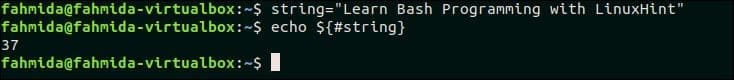### Example-2: Using `expr` to count the length of a string

Another way to count the length of a string is to use `expr` command with length keyword. The following commands will assign a value to the variable, \$string, store the length value to the variable, \$len and print the value of \$len.

\$ string="Hypertext Markup Language"
\$ len=`expr length "\$string"`
\$ echo "The length of string is \$len"

Output:

The following output will appear after running the above command.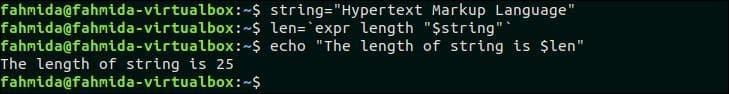Create a bash file named “len1.sh” and add the following script. Here, a string value will be taken from the user and the length of the string value will be counted by using `expr` command that will be printed later.

len1.sh

#!/bin/bash
echo  “Enter a string:”
len=`expr "\$strval" : '.*'`
echo "The length of the input string is \$len"

Run the script.

\$ bash len1.sh

Output:

Here, “I like Programming” is taken as input and the length of the string is 18.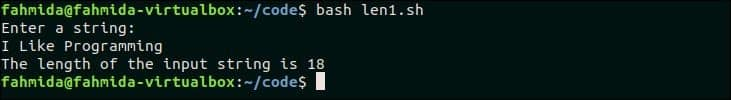### Example-3: Using `wc` to count the length of the string

Create a bash file named “len2.sh” and add the following script. This script will read the first command-line argument into the variable \$strval and count the length of \$strval by using `wc` command that will be printed later.

len2.sh

#!/bin/bash
strval=\$1
len=`echo \$strval | wc -c`
echo "The length of the first command-line argument is \$len"

Run the script with one command-line argument.

\$ bash len2.sh “Hello World”

Output:

The length of “Hello World” is 12 that is printed as output.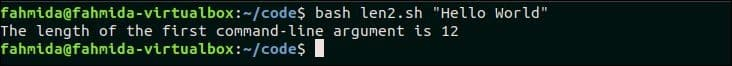### Example-4: Using `awk` to count the length of string

Create a bash file named “len3.sh” and add the following script. Here, the username will be taken as input and check the length of \$username is less than 6 or not. If the length is less than 6 then the output will “Invalid username” otherwise the output will “Valid username”.

len3.sh

#!/bin/bash
if [ \$len -lt 6 ]; then
else
fi

Run the script.

\$ bash len3.sh

Output:

Here, when “fahmida” is taken as the username then it is valid and when “lily” is taken as the username then it is invalid.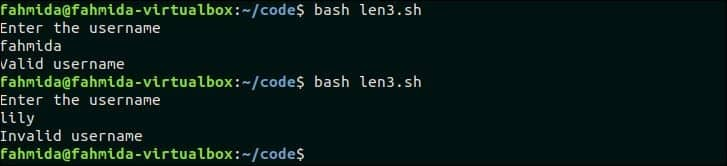#### Conclusion:

Different ways of counting the length of a string in bash are shown in this tutorial by using various examples. The user can apply any of the mentioned ways to find out the length of the string.